# Finding the missing length in a figure Online Quiz

#### Complete Python Prime Pack

9 Courses     2 eBooks

#### Artificial Intelligence & Machine Learning Prime Pack

6 Courses     1 eBooks

#### Java Prime Pack

9 Courses     2 eBooks

Following quiz provides Multiple Choice Questions (MCQs) related to Finding the missing length in a figure. You will have to read all the given answers and click over the correct answer. If you are not sure about the answer then you can check the answer using Show Answer button. You can use Next Quiz button to check new set of questions in the quiz.Q 1 - Find the missing length(s) in the following figure.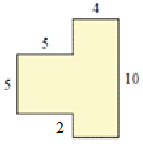### Explanation

Step 1:

Missing length = 10 – 5 – 2 = 3 units

Step 2:

Figure with missing length shown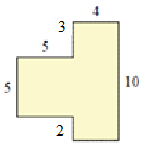Q 2 - Find the missing length(s) in the following figure.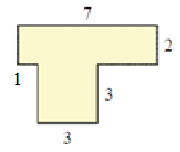### Explanation

Step 1:

Missing length = 7 – 1 – 3 = 3 units

Step 2:

Figure with missing length shown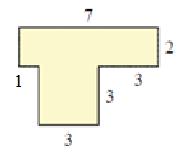Q 3 - Find the missing length(s) in the following figure.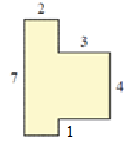### Explanation

Step 1:

Missing length = 7 – 1 – 4 = 2 units

Step 2:

Figure with missing length shown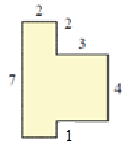Q 4 - Find the missing length(s) in the following figure.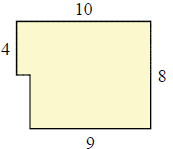### Explanation

Step 1:

Missing length = 8 – 4 = 4 units

Missing length = 10 – 9 = 1 unit

Step 2:

Figure with missing lengths shown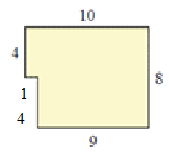Q 5 - Find the missing length(s) in the following figure.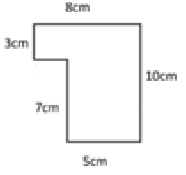### Explanation

Step 1:

Missing length = 8 – 5 = 3 cm

Step 2:

Figure with missing length shown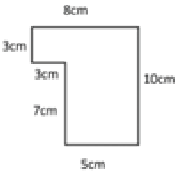Q 6 - Find the missing length(s) in the following figure.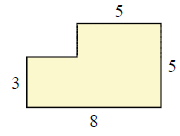### Explanation

Step 1:

Missing length = 8 – 5 = 3 units

Missing length = 5 – 3 = 2 units

Step 2:

Figure with missing lengths shown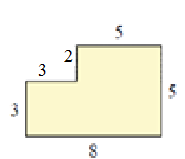Q 7 - Find the missing length(s) in the following figure.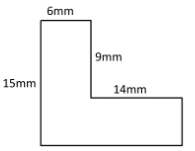### Explanation

Step 1:

Missing length = 15 – 9 = 6 mm

Missing length = 6 + 14 = 20 mm

Step 2:

Figure with missing lengths shown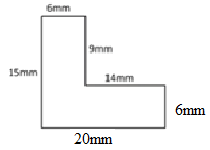Q 8 - Find the missing length(s) in the following figure.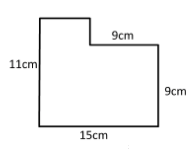### Explanation

Step 1:

Missing length = 15 – 9 = 6 cm

Missing length = 11 – 9 = 2 cm

Step 2:

Figure with missing lengths shown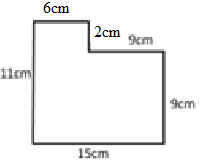Q 9 - Find the missing length(s) in the following figure.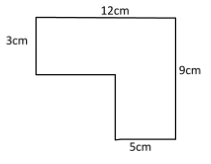### Explanation

Step 1:

Missing length = 12 – 5 = 7 cm

Missing length = 9 – 3 = 6 cm

Step 2:

Figure with missing lengths shown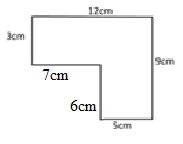Q 10 - Find the missing length(s) in the following figure.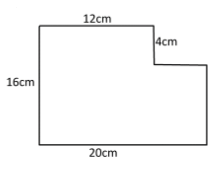### Explanation

Step 1:

Missing length = 20 – 12 = 8 cm

Missing length = 16 – 4 = 12 cm

Step 2:

Figure with missing lengths shown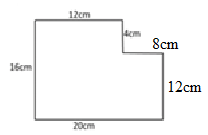finding_missing_length_in_figure.htm